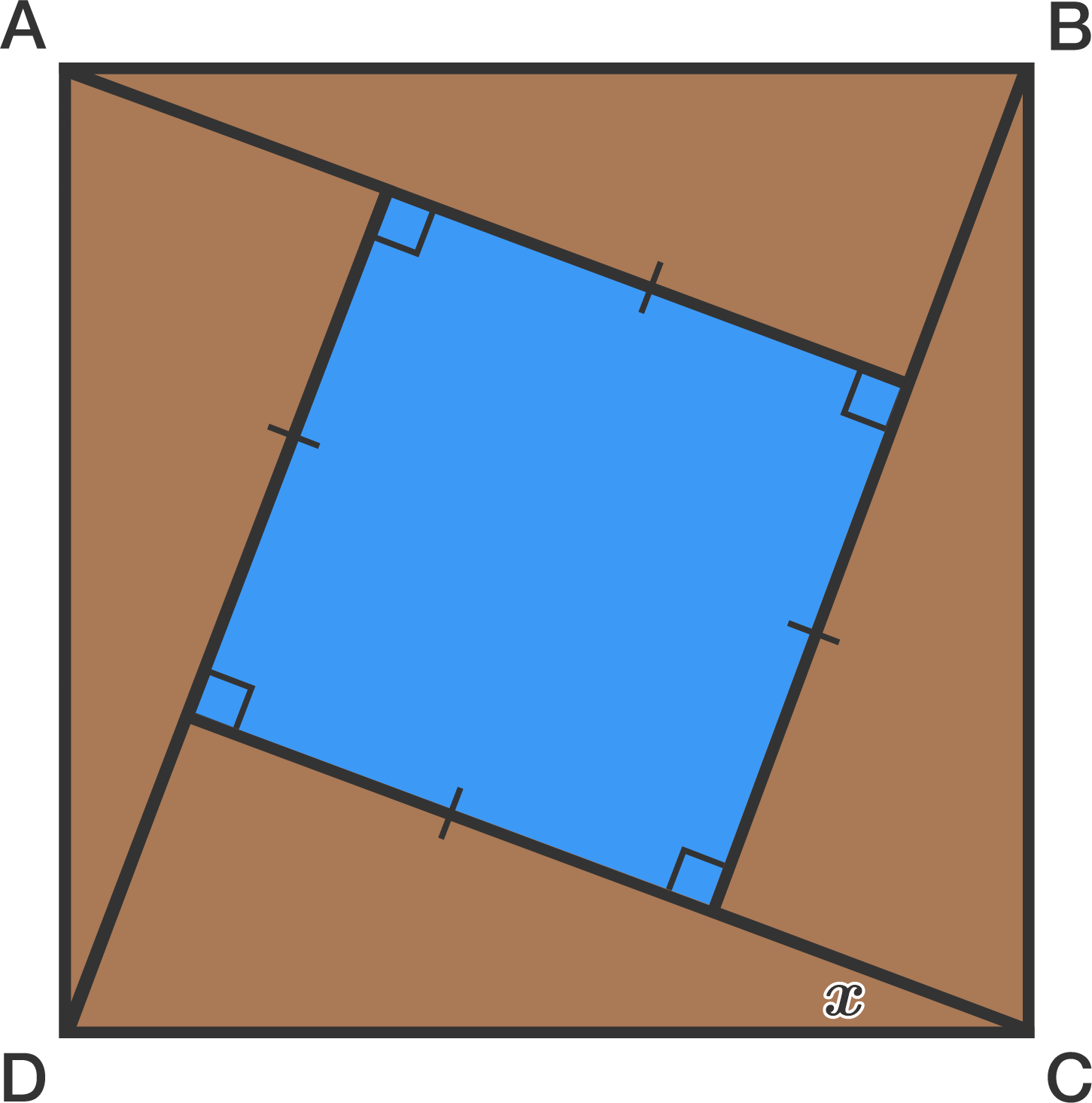2 squares

Geometry Level 3Let $ABCD$ be a square. The area of the blue square is $\dfrac12$ of the area of $ABCD$.

Find the angle $x$ in degrees.

×